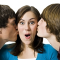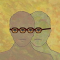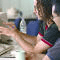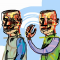Do you like the article?
Share it with others -

Use new possibilities of MetaTrader 5

# Trading Strategy Based on Pivot Points Analysis

11 October 2007, 10:100
9 251

### Introduction

Pivot Points (PP) analysis is one of the simplest and most effective strategies for high intraday volatility markets. It was used as early as in the precomputer times, when traders working at stocks could not use any ADP equipment, except for counting frames and arithmometers. Analysis of this kind can often be found in a number of articles on technical analysis in the sections devoted to excursions into history. The main advantage of this technique is its computational efficiency that allows traders to make calculations mentally or on a sheet of paper.

Since four arithmetic operations are used in calculations, every trader using this technique always wanted either outrun the competitors or, at least, "outcalculate" them. Correspondingly, there are many formulas to calculate pivot points and support/resistance levels (see examples in the table below).

 Range Possible Formulas to Calculate PP RANGE: High - Low RANGE %: (High - Low) / (Previous Close) PP1=(H+L+C)/3 PP2=(H+L+O)/3 PP3=(H+L+C+O)/4 PP4=(H+L+C+C)/4 PP5=(H+L+O+O)/4 PP6=(H+L)/2 PP7=(H+C)/2 PP8=(L+C)/2 Change Change: Close - Previous Close Change %: (Close - Previous Close) / (Previous Close) Trend % Calculation: ABS (CLOSE - OPEN) / RANGE

 Classic Formula Woodie Pivot Points R4 = R3 + RANGE (same as: PP + RANGE * 3) R3 = R2 + RANGE (same as: PP + RANGE * 2) R2 = PP + RANGE R1 = (2 * PP) - LOW PP = (HIGH + LOW + CLOSE) / 3 S1 = (2 * PP) - HIGH S2 = PP - RANGE S3 = S2 - RANGE (same as: PP - RANGE * 2) S4 = S3 - RANGE (same as: PP - RANGE * 3) R4 = R3 + RANGE R3 = H + 2 * (PP - L) (same as: R1 + RANGE) R2 = PP + RANGE R1 = (2 * PP) - LOW PP = (HIGH + LOW + CLOSE) / 3 S1 = (2 * PP) - HIGH S2 = PP - RANGE S3 = L - 2 * (H - PP) (same as: S1 - RANGE) S4 = S3 - RANGE Camarilla Pivot Points Tom DeMark "Pivot Points" R4 = C + RANGE * 1.1/2 R3 = C + RANGE * 1.1/4 R2 = C + RANGE * 1.1/6 R1 = C + RANGE * 1.1/12 PP = (HIGH + LOW + CLOSE) / 3 S1 = C - RANGE * 1.1/12 S2 = C - RANGE * 1.1/6 S3 = C - RANGE * 1.1/4 S4 = C - RANGE * 1.1/2 R1 = X / 2 - L PP = X / 4 (this is not an official DeMark number but merely a reference point based on the calculation of X) S1 = X / 2 - H Condition if Open after Close if C < O then X = (H + (L * 2) + C) if C > O then X = ((H * 2) + L + C) if C = 0 then X = (H + L + (C * 2))

### Problems and Disappointments

In the world of probability Forex also relates to, finding of a Pivot Point with unique computing result is something like an oasis in a desert. This unambiguity and simplicity of arithmetics attract novice traders.

However, this notorious unambiguity is the result of arithmetic operations and has no relation to Forex. The duality of this situation irritates, in case the results of calculations performed by traders using data from different DataCenters are different. The differences between the results and the forecasts of analyst Rudolph Axel, the acknowledged leader in Pivot techniques application, are even more irritating. Let us try to separate the husk from the grain.

### Not All Roses

To generate a pivot point and support/resistance levels for a future period of time, Pivot Points Analysis uses the minimal amount of inputs: High, Low and Close of the preceding tick period. Initially, such period was a trading session.

Far back in the past, when the main rules of Pivot and support/resistance levels were developed, a "trading session" and a "trading day" were perhaps the same. At present, the trading day time in forex consists of three main trading sessions, so attempts to use the rules of the Pivot Points Analysis without taking these change into consideration are not quite correct. Time is the parameter that is present in trading, but not shown in calculating formulas. In our topic under consideration, it determines High, Low and Close of the period used in calculations. This is the first "thorn" in the idea.

### Another "Thorn"

It's the terminal's internal time. Instead of being the same (GMT) in all the terminal, it is different in different Data Centers. This results in an interesting effect: the time within which a candlestick is formed is the same for only timeframes smaller than H1, then there are divergences observed. So the analysis, or its reliability and unambiguity on charts of different Data Centers, is open to question.

To exclude the situation when the internal terminal time influences calculations, it is necessary to use one-hour candlesticks corrected for the difference between the terminal time and GMT.
This thorn can be eliminated using indicator DailyPivot_Shift (https://www.mql5.com/ru/code/8864). Indicator DailyPivot_Shift differs from the normal indicator DailyPivot through that the basic levels can be calculated with a shift in relation to the beginning of the day. Thus, the levels can be calculated on the basis of local time, not the server time, for example, GMT. As well, the indicator does not consider information about weekend's quotes when it builds charts on Mondays.

### The Third "Thorn"

We want to use one-hour candlesticks, but we can only get them in the manual mode, separately for each currency pair. The author does not mean an advanced programmer, but, for example, a physician or an economist.

This means that the time needed for analyzing will be wasted on nonproductive manual operations.

### On Computational Accuracy

The table below gives absolute values of Pivot levels at different values of Close, and the absolute value of deviations in points.

 Calculation of Pivot levels at different values of Close -30 -10 0 10 30 GBPUSD GBPUSD GBPUSD GBPUSD GBPUSD R3 1,8566 1,8580 1,8586 1,8593 1,8606 R2 1,8524 1,8530 1,8534 1,8537 1,8544 R1 1,8450 1,8464 1,8470 1,8477 1,8490 Pivot 1,8408 1,8414 1,8418 1,8421 1,8428 S1 1,8334 1,8348 1,8354 1,8361 1,8374 S2 1,8292 1,8298 1,8302 1,8305 1,8312 S3 1,8218 1,8232 1,8238 1,8245 1,8258 H 1,8481 1,8481 1,8481 1,8481 1,8481 L 1,8365 1,8365 1,8365 1,8365 1,8365 C 1,8377 1,8397 1,8407 1,8417 1,8437 -30 -10 0 10 30

 deviations of the mean value, in points * GBPUSD GBPUSD GBPUSD GBPUSD GBPUSD R3 -20 -6,7 1,8586 6,7 20 R2 -10 3,3 1,8534 3,3 10 R1 -20 6,7 1,8470 6,7 20 Pivot -10 3,3 1,8418 3,3 10 S1 -20 6,7 1,8354 6,7 20 S2 -10 3,3 1,8302 3,3 10 S3 -20 6,7 1,8238 6,7 20 H 1,8481 1,8481 1,8481 1,8481 1,8481 L 1,8365 1,8365 1,8365 1,8365 1,8365 C 1,8377 1,8397 1,8407 1,8417 1,8437

Deviation of the period close price (or the summarized deviation of H+L+C) by 30 points results in the error of 10 points.

### Quick Calculation

The classical formula is as follows: PP = (HIGH + LOW + CLOSE) / 3

A variation looks like this: PP = (H + L) / 2

Suppose H = 1.9100, L = 1.9000, Range = 100. Then, by definition, "Close" must be within the range of 1.9000 – 1.9100.

 High Low Close (H+L+C)/3 (H+L)/2 /3 -'/2 1.9100 1.9000 1.9000 1.9033 1.9050 -17 1.9100 1.9000 1.9010 1.9037 1.9050 -13 1.9100 1.9000 1.9020 1.9040 1.9050 -10 1.9100 1.9000 1.9030 1.9043 1.9050 -7 1.9100 1.9000 1.9040 1.9047 1.9050 -3 1.9100 1.9000 1.9050 1.9050 1.9050 0 1.9100 1.9000 1.9060 1.9053 1.9050 3 1.9100 1.9000 1.9070 1.9057 1.9050 7 1.9100 1.9000 1.9080 1.9060 1.9050 10 1.9100 1.9000 1.9090 1.9063 1.9050 13 1.9100 1.9000 1.9100 1.9067 1.9050 17

We can see that the deviation of the close price from (H+L)/2 up to 30 points results in an error within 10 points. It means that if the movement has not started and the price does not break High and Low levels, stays somewhere in the middle of the range, we get a PP using Andrews' Pitchfork directly in the chart, whereas deviations are within 10 points from Axel's data. Moreover, I didn't do it myself due to absence Axel's archives. Something may be done through searching in Axel's Forecast and (H+L+C)/3, (H+L)/2 (on the preceding session).

### Support/Resistance Levels

The formulas are given above. It is necessary to avoid the erroneous assumption about comprehension unambiguity of calculation results, such as R3 = 1. 9356, nothing more or less than that, and accept the following calculation order. The support/resistance level calculation result is accurate up to the nearest support/resistance level of the real history in the chart. This is what Rudolph Axel demonstrates us, actually.

Example: "The intraday EURJPY: The pair is traded near the minor resistance of 158. 38 (maximum of the 9th of February). If this level is broken through, the pair will aim at 158.76 (maximum of the 14th of February). The minor support is located near 157.78 (minimum of Wednesday) and at 157.28."

### Conclusion

I haven't found any theory that would prove or disprove the Pivot Point or Support/Resistant Levels calculation results. We will use the generalized, accepted by practically all scientists rules: "Practice is the sole criterion of truth". Experienced traders in all around the world consider these reference values to be rather close to the truth with rather high statistical advantage over coin tossing.

One may dispute the usability, correctness and accuracy of these calculations as long and well-reasoned as one like. However, simple formulas and monotonicity of their usage allow a trader of any level to gain experiences and find legs. "Begin at the beginning."

P.S. The article was prepared by Moderator of forum www.forum.profiforex.ru, Vladimir aka dedd.

Translated from Russian by MetaQuotes Software Corp.
Original article: https://www.mql5.com/ru/articles/1465Automated Choice of Brokerage Company for an Efficient Operation of Expert Advisors

It is not a secret that for an efficient operation of Expert Advisors we need to find a suitable brokerage company. This article describes a system approach to this search. You will get acquainted with the process of creating a program with dll for working with different terminals.MT4TerminalSync - System for the Synchronization of MetaTrader 4 Terminals

This article is devoted to the topic "Widening possibilities of MQL4 programs by using functions of operating systems and other means of program development". The article describes an example of a program system that implements the task of the synchronization of several terminal copies based on a single source template.Object Approach in MQL

This article will be interesting first of all for programmers both beginners and professionals working in MQL environment. Also it would be useful if this article were read by MQL environment developers and ideologists, because questions that are analyzed here may become projects for future implementation of MetaTrader and MQL.Terminal Service Client. How to Make Pocket PC a Big Brother's Friend

The article describes the way of connecting to the remote PC with installed MT4 Client Terminal via a PDA.